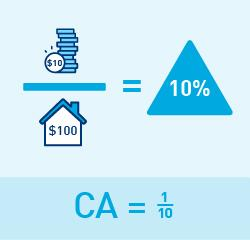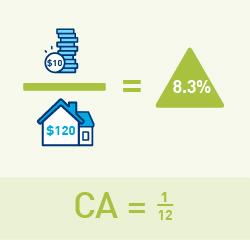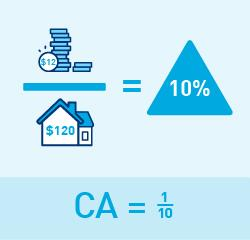# APRA Explains: Risk-weighted assets

In order to protect depositors from the risk of a bank becoming insolvent, APRA requires banks and other authorised deposit taking institutions to fund themselves with a minimum amount of capital. To determine the minimum amount of capital that banks must have, APRA sets minimum capital adequacy ratios.

The capital adequacy ratio is calculated as eligible capital divided by risk-weighted assets.

Risk-weighted assets, or RWA, are used to link the minimum amount of capital that banks must have, with the risk profile of the bank’s lending activities (and other assets). The more risk a bank is taking, the more capital is needed to protect depositors.

In the last edition of APRA Insight, we explained what constitutes eligible capital i.e. the numerator of the capital adequacy ratio. In this edition, we focus on the ratio’s denominator i.e. risk-weighted assets.

Essentially, risk-weighted assets are the loans and other assets of a bank, weighted (that is, multiplied by a percentage factor) to reflect their respective level of risk of loss to the bank. For example, mortgages secured by residential property are generally considered to be lower risk than unsecured credit card lending. As such, the greater the amount of higher risk assets and loans that a bank has, the higher its risk-weighted assets, and therefore, the higher the amount of capital the bank must have in order to meet APRA’s minimum capital adequacy ratios.

Australia’s capital adequacy framework for banks is based on the internationally agreed Basel framework, with some modifications to better tailor the framework to Australian risks. The major risk reflected in capital adequacy ratios for banks is credit risk – that is, the risk of loan losses – but other risks such as those relating to operational risks and market price movements are also captured.

## How risk-weighted assets are calculated for credit risk

Risk-weighted assets are designed to be responsive to changes in both the quality and composition of a bank’s lending portfolio. In an economic downturn, as credit risk increases, risk-weighted assets would also be expected to increase to some extent.

There are two broad approaches to calculating credit risk-weighted assets:

1. The standardised approach, and
2. The internal ratings-based (IRB) approach.

Typically, only the larger banks have the expertise and infrastructure necessary to use the IRB approach. However, under both approaches, risk-weighted assets are broadly calculated as the risk weight multiplied by the exposure amount.

### Risk weights

Risk weights are essentially percentage factors that adjust for the credit risk of different types of assets. Under the standardised approach, banks must apply APRA-prescribed risk weights. For example, cash and Australian Government securities are considered to represent a lower risk (zero per cent risk weight) than an unsecured loan to a business (100 per cent risk weight). Under the IRB approach, banks are allowed to use APRA-approved models to calculate risk estimates (for example, the probability of default) to assess the credit risk of borrowers. These risk estimates are then used as inputs in APRA-prescribed formulas.

### Exposure amount

The exposure amount refers to the total amount that a bank could potentially lose if a borrower defaults. This includes not only the funds actually advanced by a bank (the drawn or on-balance sheet amount), but also any additional amounts up to advised limits that the bank may be contractually obliged to advance in the future (undrawn or off-balance sheet amount).

To calculate the on-balance sheet equivalent for off-balance sheet amounts, a bank multiplies the undrawn amount by a credit conversion factor (which represents the likelihood of a bank being required to advance additional funds).

### Calculating risk-weighted assets

Banks calculate risk-weighted assets by multiplying the exposure amount by the relevant risk weight for the type of loan or asset. A bank repeats this calculation for all of its loans and assets, and adds them together to calculate total credit risk-weighted assets.

The example below assumes that a bank has total assets worth \$100:

#### RWA (\$)

Cash

\$20

0%

\$0

Australian Government securities

\$10

0%

\$0

Housing loans

\$40

35%

\$14

\$30

100%

\$30

Total

\$100

N/A

\$44

The total credit risk-weighted assets (\$44 in the example above) are added to risk-weighted assets for operational risk and market risk, and used as the denominator in the capital adequacy ratio.

The higher a bank’s total risk-weighted assets, the more capital it will need in order to meet the minimum capital adequacy ratios set by APRA. For example:

Assume a bank has \$10 of capital and seeks to have a 10% capital ratio. Then the bank can have \$100 of risk-weighted assets. (Figure 1)

If the bank’s risk-weighted assets grow to \$120, then its capital ratio will fall to 8.3%. (Figure 2)

So if the bank wishes to maintain a 10% capital ratio, it will need to raise an extra \$2 of capital. (Figure 3)Figure 1Figure 2Figure 3

The risk-weighted assets calculation therefore plays an important role in ensuring that the capital held by banks corresponds with their overall risk profile.

### Media enquiries

Contact APRA Media Unit, on +61 2 9210 3636